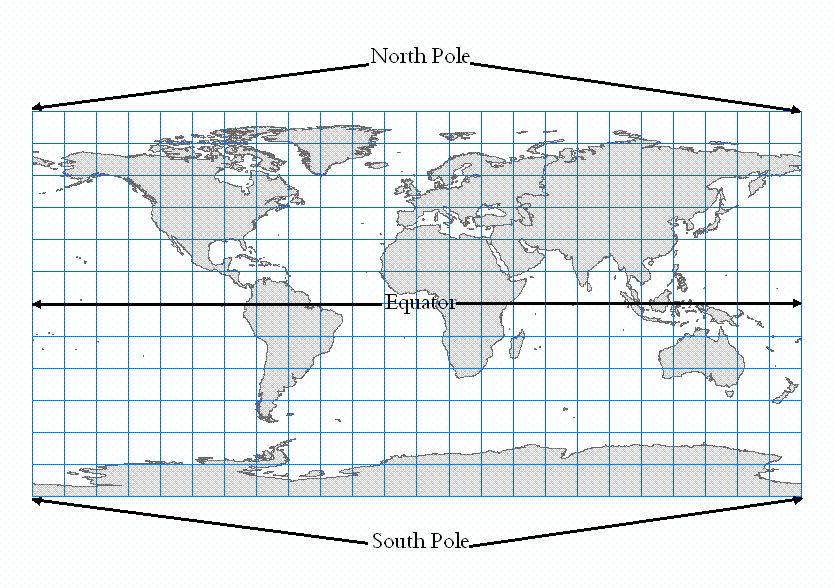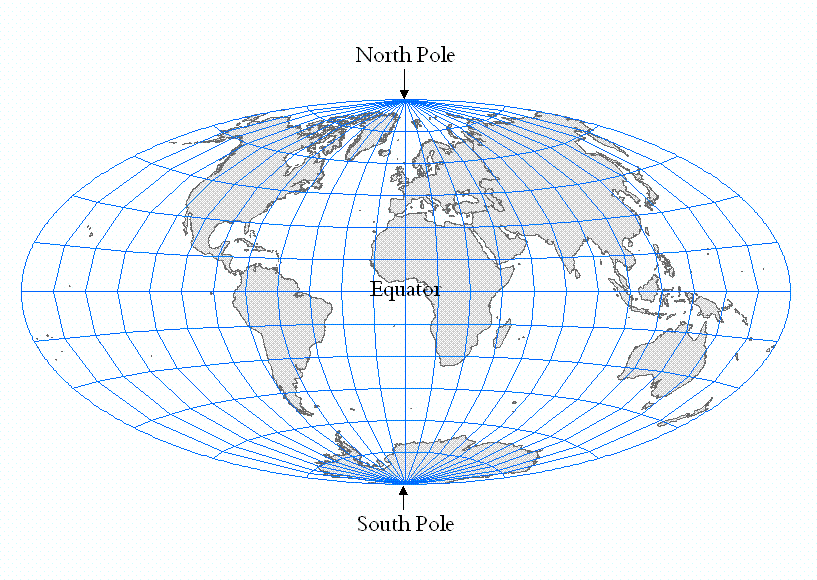English

# FAQ: Why does the scale bar measure incorrectly when the ArcMap Data Frame is in a Geographic Coordinate System?

## Question

Why does the scale bar measure incorrectly when the ArcMap Data Frame is in a Geographic Coordinate System?

A scale bar on a map that is set to a Geographic Coordinate System, using units of decimal degrees, only measures distances correctly at the Equator.

To apply a scale bar that measures distances correctly over all areas of the Earth's surface, the ArcMap Data Frame must be set to a Projected Coordinate System.

A scale bar measures linear units, such as feet, meters, kilometers, and miles.

When the ArcMap Data Frame is set to a Geographic Coordinate System, the units of measure are angular units. The number of degrees in the angle formed from the prime meridian at Greenwich, England to the center of the Earth, then back to the location of the data is the number of degrees, minutes and seconds of longitude (the X coordinate). The number of degrees formed in the angle from the Equator, to the center of the Earth then up or down to the location of the data is the number of degrees, minutes and seconds of latitude (the Y coordinate).

At the Equator, a degree of longitude measures about 69 miles, but at the North or South Pole, the length of a degree of Longitude is 0.

As the lines of longitude converge toward the North or South Pole, the measured distance between these lines of longitude across the surface of the Earth becomes shorter and shorter, while the angular measurement stays the same.

The following image shows a view of the surface of the Earth displayed in a Geographic Coordinate System. Notice that this distorts the North and South Poles, which are points, and displays them as lines which appear as long as the Equator.The following image shows a view the surface of the Earth, displayed in a Projected Coordinate System, in this case, Aitoff. Notice that the North and South Poles are correctly displayed as points.For help selecting a suitable coordinate system refer to the article 'Select a suitable map projection or coordinate system' in the Related Information section below.

## Related Information

Last Published: 3/12/2019

Article ID: 000007211

Software: ArcGIS-ArcEditor 9.3.1, 9.3, 9.2, 10 ArcGIS-ArcInfo 9.3.1, 9.3, 9.2, 10 ArcGIS-ArcView 9.3.1, 9.3, 9.2, 10